# Point-Slope Form

An easy way to write the equation of a line if we know the slope and a point on a line.

This post is part of a series.

Suppose we want to write the equation of a line with a given slope $m=2$, through a particular point $(3,5)$. In the previous post, we substituted the given information into a slope-intercept equation form $y=mx+b$, solved for $b$, and rewrote the slope-intercept form with $m$ and $b$ substituted so that $x$ and $y$ were the only variables.

\begin{align*} \text{Slope-intercept equation form} \hspace{.5cm} &\Bigg| \hspace{.5cm} y=mx+b \\ \text{Substitute the given slope } m=2 \hspace{.5cm} &\Bigg| \hspace{.5cm} y=2x+b \\ \text{Substitute the given point } (3,5) \hspace{.5cm} &\Bigg| \hspace{.5cm} 5=2(3)+b \\ \text{Solve for } b \hspace{.5cm} &\Bigg| \hspace{.5cm} b=-1 \\ \text{Final equation} \hspace{.5cm} &\Bigg| \hspace{.5cm} y=2x-1 \end{align*}

However, there is an alternative form, point-slope form, that makes it even easier to write the equation of a line if we know the slope and a point $(x_0,y_0)$ on the line. It is given by

$y-y_0 = m(x-x_0).$

If we know that our desired line has slope $m=2$ and passes through the point $(x_0,y_0)=(3,5)$, then we can substitute directly into point-slope form without performing any additional computations:

$y-5 = 2(x-x3)$

This is an accepted form of the equation for a line, so we don’t need to simplify it at all unless we’re asked to do so.

But even if we actually need to find the line in slope-intercept form, it’s still advantageous to begin with point-slope form, because all we have to do is distribute the $2$ and add $5$ to get to slope-intercept form.

\begin{align*} \text{Point-slope form} \hspace{.5cm} &\Bigg| \hspace{.5cm} y-5=2(x-3) \\ \text{Distribute the } 2 \hspace{.5cm} &\Bigg| \hspace{.5cm} y-5=2x-6 \\ \begin{matrix} \text{Add } 5 \text{ to both sides to reach} \\ \text{slope-intercept form} \end{matrix} \hspace{.5cm} &\Big| \hspace{.5cm} y=2x-1 \end{align*}

## Derivation

The point-slope formula is easy to remember, too, because it just says that the slope between any point $(x,y)$ and the reference point $(x_0,y_0)$ needs to be equal to the given slope $m$.

Moving from $(x_0,y_0)$ to $(x,y)$, the amount we go up is $y-y_0$, and the amount we go over horizontally is $x-x_0$, so the slope is just $\frac{y-y_0}{x-x_0}$. Equating this to $m$ and multiplying to get rid of the fraction, we reach point-slope form!

\begin{align*} \text{Slope must equalm} \hspace{.5cm} &\Bigg| \hspace{.5cm} \dfrac{y-y_0}{x-x_0} = m \\ \begin{matrix} \text{Multiply both sides by } x-x_0 \\ \text{to reach point-slope form} \end{matrix} \hspace{.5cm} &\Bigg| \hspace{.5cm} y-y_0=m(x-x_0) \end{align*}

## Graphing

To graph a line whose equation is given in point-slope form, we perform the same process as we do to graph a line that is in slope-intercept form, except we start at the reference point rather than at the y-intercept.

For example, consider the line $y-4 = \frac{3}{2}(x-1)$, for which the reference point is $(1,4)$ and the slope is $\frac{3}{2}$. To graph this line, we start at $(1,4)$, go up $3$ and over $2$ to the point $(3,7)$, and draw a line through the two points.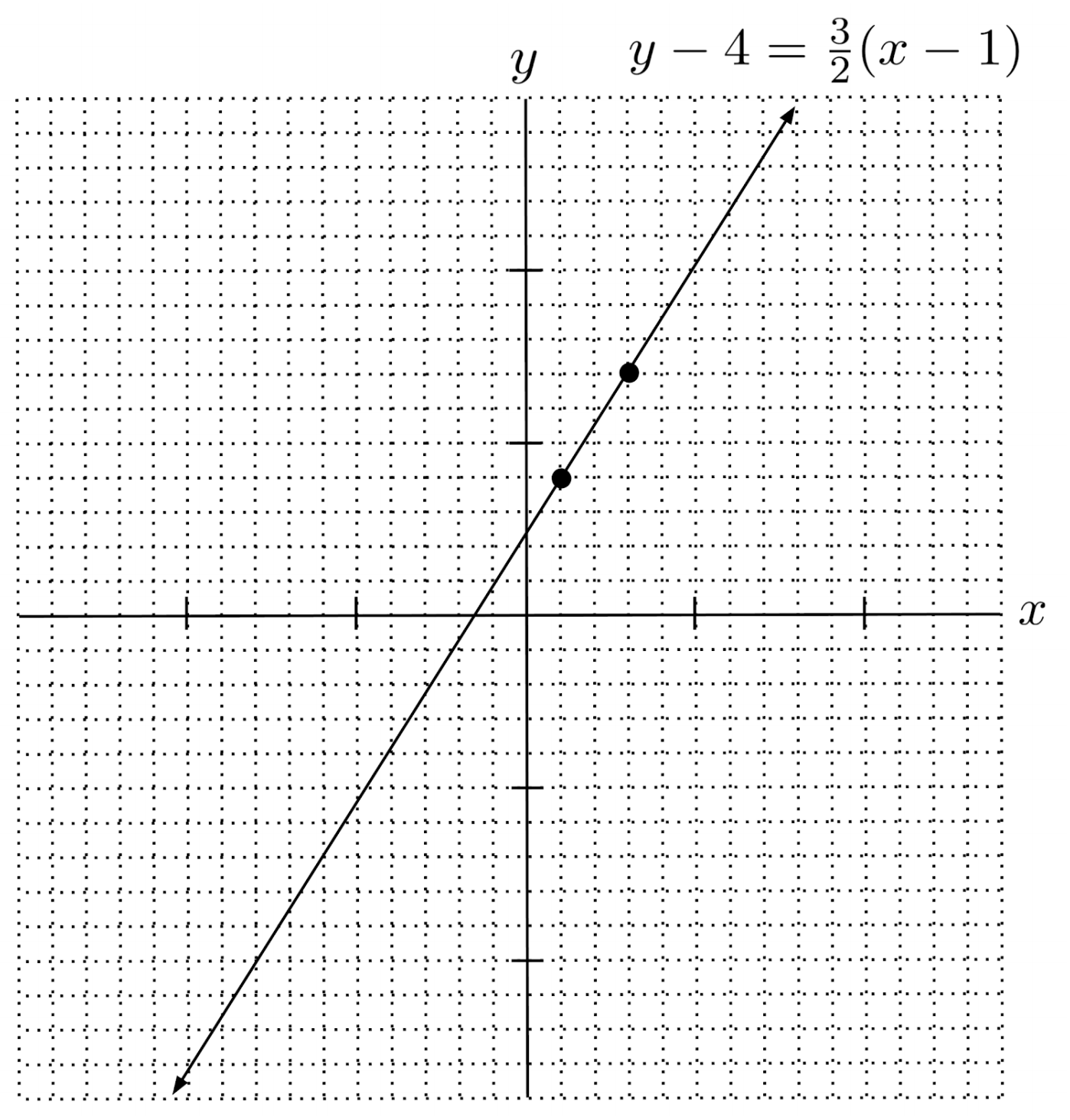## Final Remark

One thing to watch out for in point-slope form: be careful about negatives.

For example, the point-slope form of a line with slope $2$ that goes through the point $(-3,-5)$ is NOT given by $y-5=2(x-3)$. This is the line that goes through the point $(3,5)$, not $(-3,-5)$.

The line that goes through $(-3,-5)$ actually involves addition rather than subtraction, because the negatives cancel the subtraction in the original formula for point-slope form.

\begin{align*} \text{Point-slope formula} \hspace{.5cm} &\Bigg| \hspace{.5cm} y-y_0=m(x-x_0) \\ \begin{matrix} \text{Substitute slope } 2 \text{ and} \\ \text{point } (-3,-5) \end{matrix} \hspace{.5cm} &\Bigg| \hspace{.5cm} y-(-5) = 2( x-(-3) ) \\ \text{Negatives cancel} \hspace{.5cm} &\Bigg| \hspace{.5cm} y+5=2(x+3) \end{align*}

## Exercises

Write the point-slope equation of the line that goes through the given point, with the given slope. (You can view the solution by clicking on the problem.)

$1) \hspace{.5cm} (1,5) \hspace{.5cm} m=2$
Solution:
$y-5=2(x-1)$

$2) \hspace{.5cm} (-2,3) \hspace{.5cm} m=8$
Solution:
$y-3=8(x+2)$

$3) \hspace{.5cm} \left( \frac{1}{2}, 2 \right) \hspace{.5cm} m=\frac{3}{8}$
Solution:
$y+2=\frac{3}{8} \left( x- \frac{1}{2} \right)$

$4) \hspace{.5cm} \left( -\frac{4}{7},-\frac{8}{13} \right) \hspace{.5cm} m=-\frac{12}{5}$
Solution:
$y - \frac{8}{13} = -\frac{12}{5} \left( x + \frac{4}{7} \right)$

Write the point-slope equation of the line that goes through the given points. (You can view the solution by clicking on the problem.)

$5) \hspace{.5cm} (2,-1) \hspace{.5cm} (1,1)$
Solution:
$y+1=-2(x-2)$
$\text{or } y-1 = -2(x-1)$

$6) \hspace{.5cm} (1,8) \hspace{.5cm} (-4,-7)$
Solution:
$y-8=3(x-1)$
$\text{or } y+7 = 3(x+4)$

$7) \hspace{.5cm} \left( \frac{1}{3}, 3 \right) \hspace{.5cm} (1,4)$
Solution:
$y-3=\frac{3}{2}\left( x-\frac{1}{3} \right)$
$\text{or } y-4 = \frac{3}{2}(x-1)$

$8) \hspace{.5cm} \left( -\frac{3}{4}, \frac{1}{2} \right) \hspace{.5cm} \left( \frac{1}{2}, \frac{3}{4} \right)$
Solution:
$y-\frac{1}{2} = \frac{1}{5} \left( x+\frac{3}{4} \right)$
$\text{or } y-\frac{3}{4} = \frac{1}{5} \left( x-\frac{1}{2} \right)$

Graph the following lines. (You can view the solution by clicking on the problem.)

$9) \hspace{.5cm} y-2=3(x-4)$
Solution: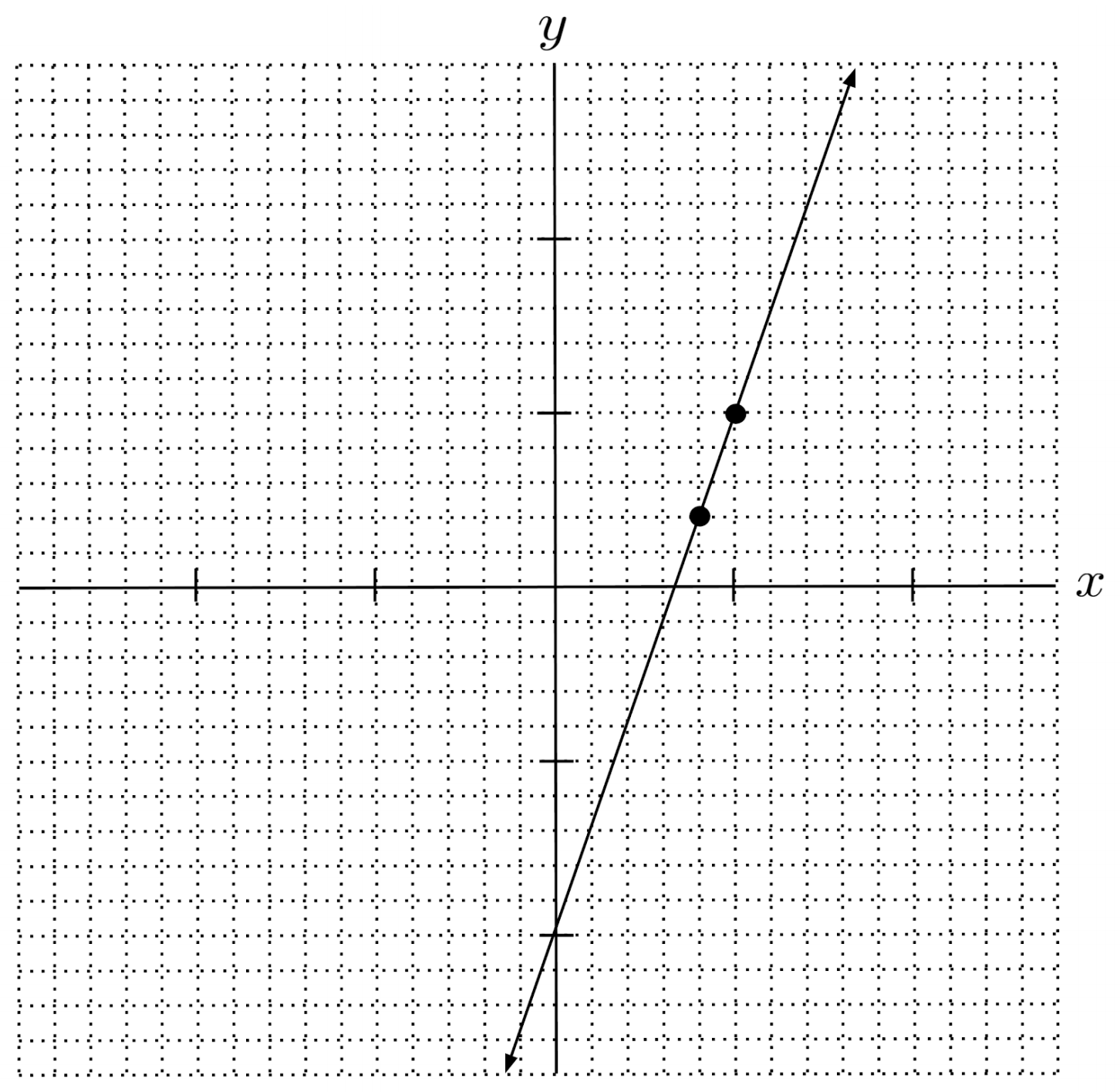$10) \hspace{.5cm} y+7=-2(x-2)$
Solution: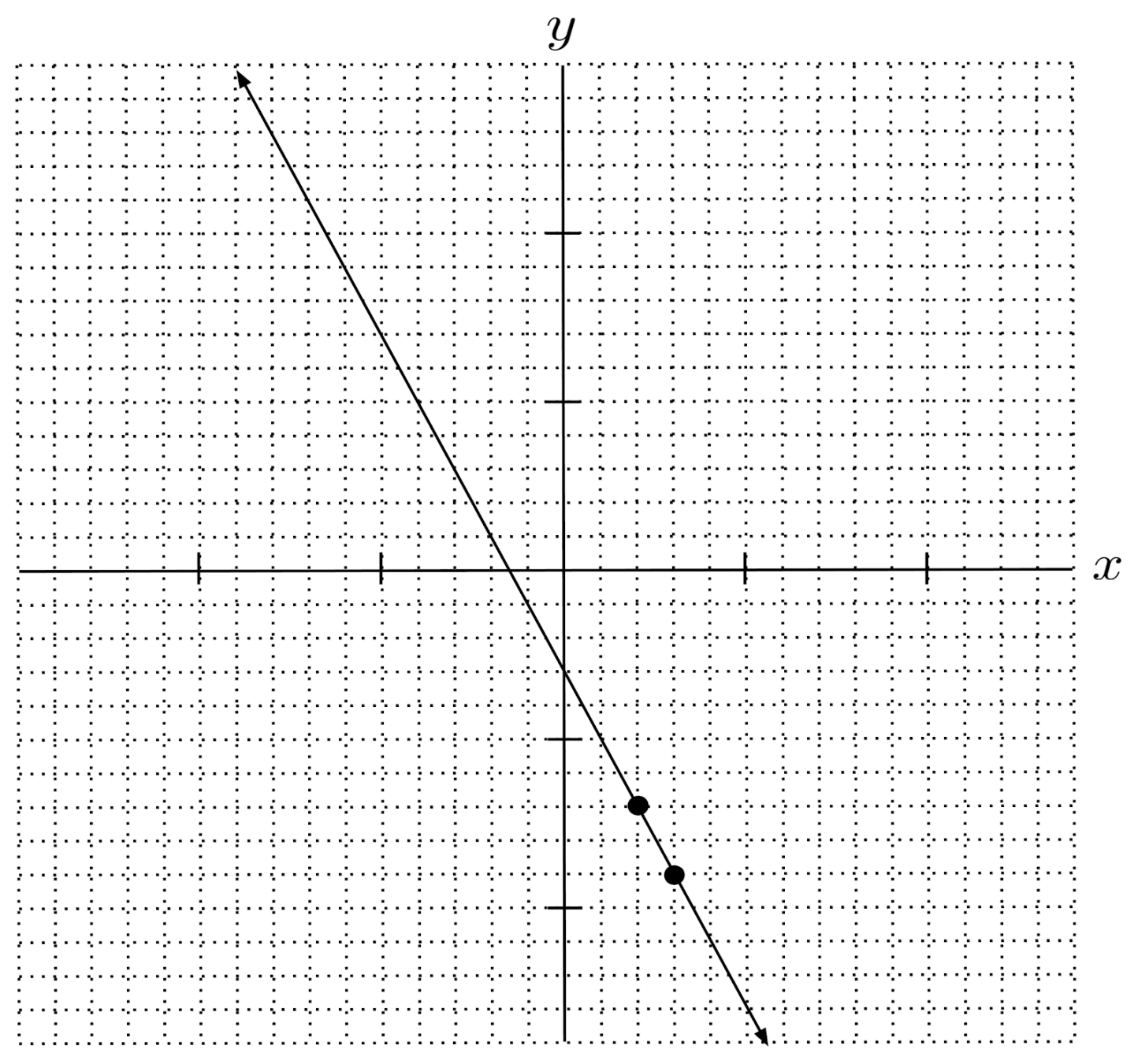$11) \hspace{.5cm} y-\frac{1}{2} = \frac{1}{3}(x+1)$
Solution: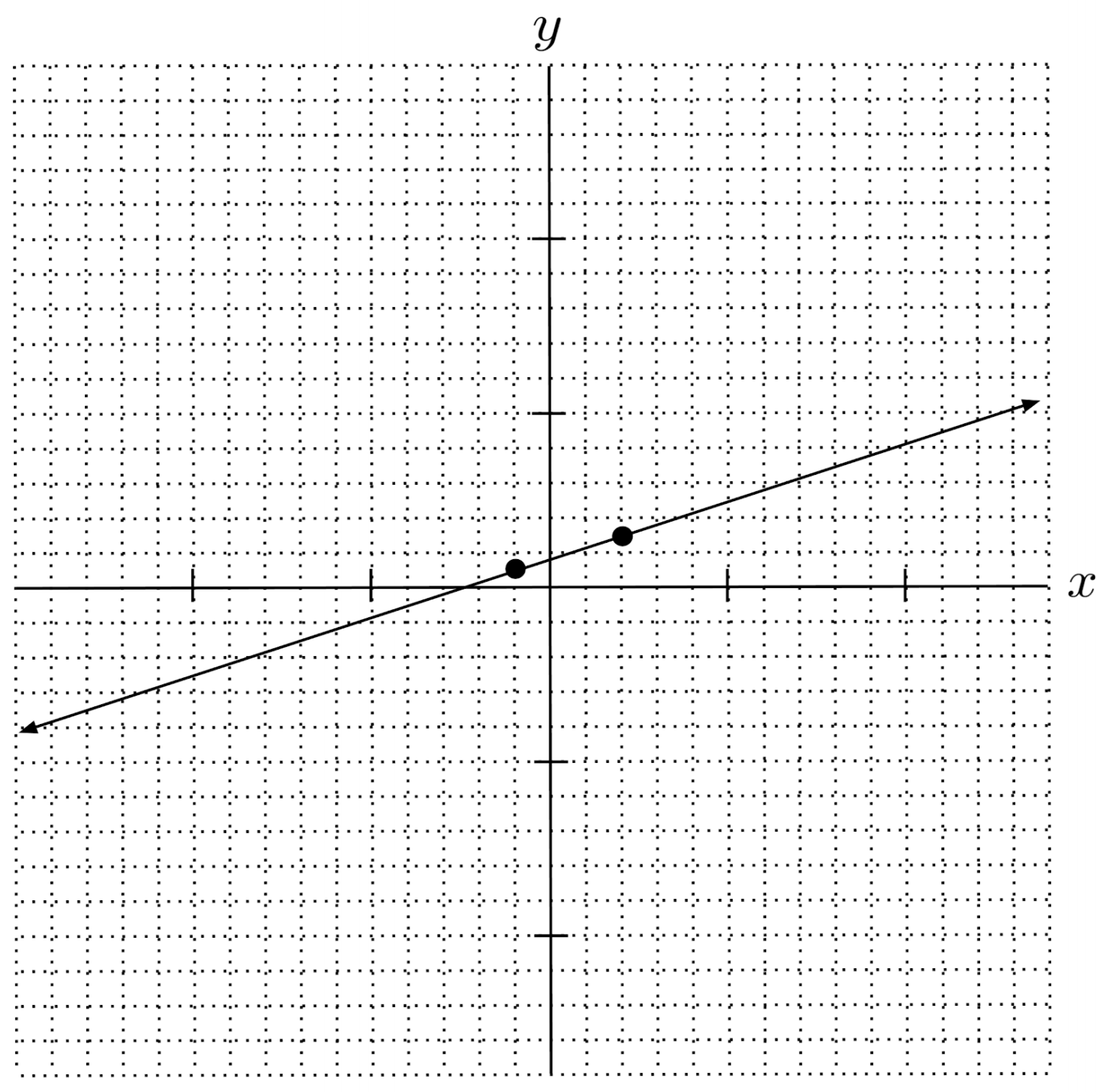$12) \hspace{.5cm} y+\frac{5}{2} = -\frac{2}{5}\left( x-\frac{1}{4} \right)$
Solution: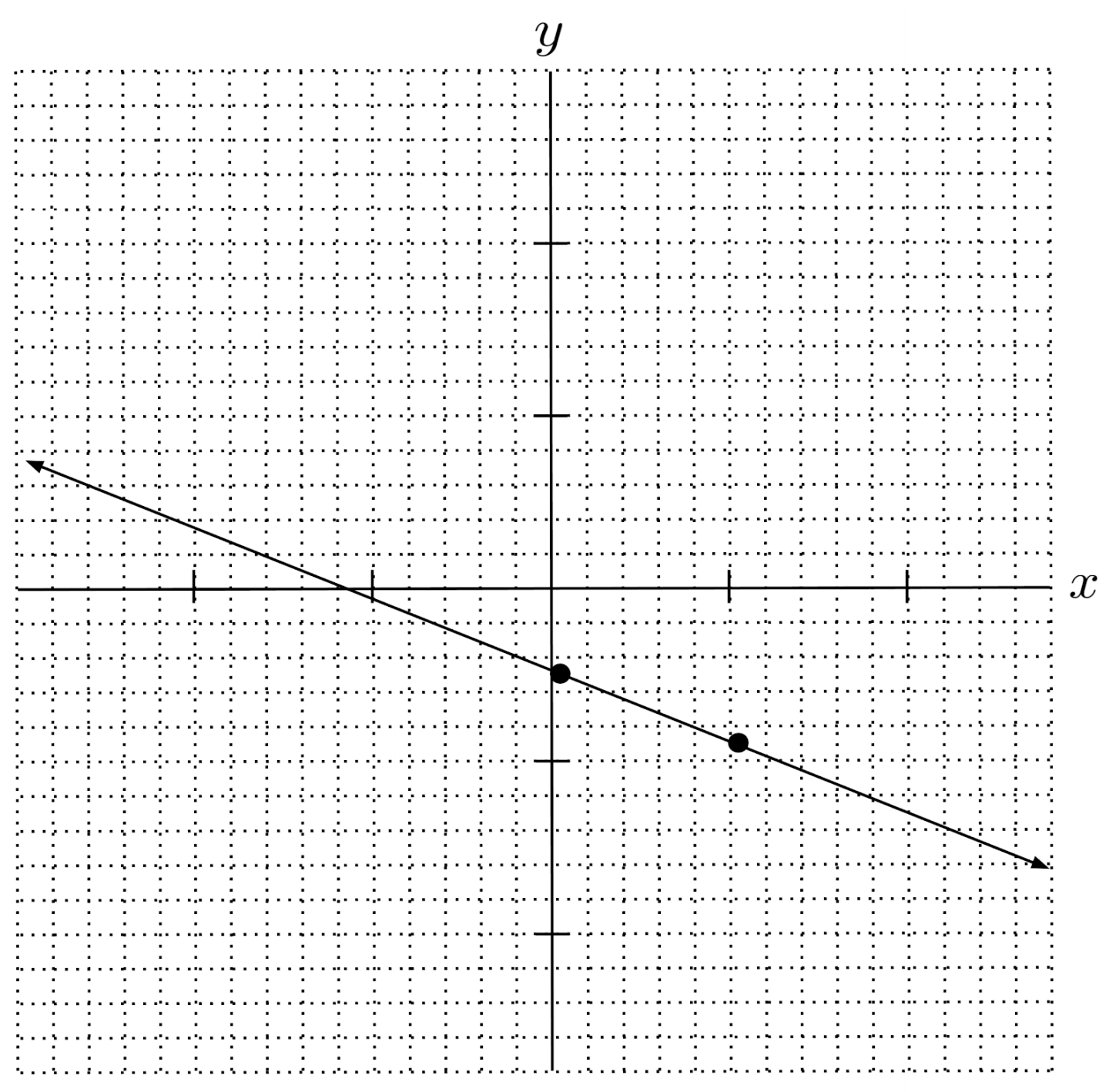This post is part of a series.